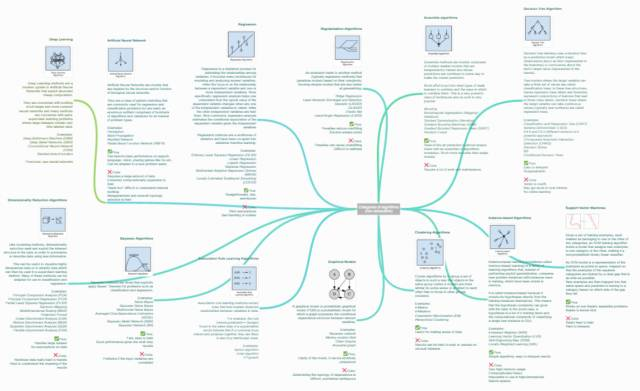# 机器学习算法集锦：从贝叶斯到深度学习及各自优缺点

2017年2月19日 126点热度 0人点赞 0条评论

https://static.coggle.it/diagram/WHeBqDIrJRk-kDDY• 正则化算法（Regularization Algorithms）

• 集成算法（Ensemble Algorithms）

• 决策树算法（Decision Tree Algorithm）

• 回归（Regression）

• 人工神经网络（Artificial Neural Network）

• 深度学习（Deep Learning）

• 支持向量机（Support Vector Machine）

• 降维算法（Dimensionality Reduction Algorithms）

• 聚类算法（Clustering Algorithms）

• 基于实例的算法（Instance-based Algorithms）

• 贝叶斯算法（Bayesian Algorithms）

• 关联规则学习算法（Association Rule Learning Algorithms）

• 图模型（Graphical Models）• 岭回归（Ridge Regression）

• 最小绝对收缩与选择算子（LASSO）

• GLASSO

• 弹性网络（Elastic Net）

• 最小角回归（Least-Angle Regression）

• 其惩罚会减少过拟合

• 总会有解决方法

• 惩罚会造成欠拟合

• 很难校准• Boosting

• Bootstrapped Aggregation（Bagging）

• 层叠泛化（Stacked Generalization）（blending）

• 随机森林（Random Forest）

• 当先最先进的预测几乎都使用了算法集成。它比使用单个模型预测出来的结果要精确的多

• 需要大量的维护工作• 分类和回归树（Classification and Regression Tree，CART）

• Iterative Dichotomiser 3（ID3）

• C4.5 和 C5.0（一种强大方法的两个不同版本）

• 容易解释

• 非参数型

• 趋向过拟合

• 可能或陷于局部最小值中

• 没有在线学习• 普通最小二乘回归（Ordinary Least Squares Regression，OLSR）

• 线性回归（Linear Regression）

• 逻辑回归（Logistic Regression）

• 逐步回归（Stepwise Regression）

• 本地散点平滑估计（Locally Estimated Scatterplot Smoothing，LOESS）

• 直接、快速

• 知名度高

• 要求严格的假设

• 需要处理异常值• 感知器

• 反向传播

• Hopfield 网络

• 在语音、语义、视觉、各类游戏（如围棋）的任务中表现极好。

• 算法可以快速调整，适应新的问题。• 深玻耳兹曼机（Deep Boltzmann Machine，DBM）

• Deep Belief Networks（DBN）

• 卷积神经网络（CNN）

• Stacked Auto-EncodersSVM 模型将训练事例表示为空间中的点，它们被映射到一幅图中，由一条明确的、尽可能宽的间隔分开以区分两个类别。

• 非常难以训练

• 很难解释• 主成分分析（Principal Component Analysis (PCA)）

• 主成分回归（Principal Component Regression (PCR)）

• 偏最小二乘回归（Partial Least Squares Regression (PLSR)）

• Sammon 映射（Sammon Mapping）

• 多维尺度变换（Multidimensional Scaling (MDS)）

• 投影寻踪（Projection Pursuit）

• 线性判别分析（Linear Discriminant Analysis (LDA)）

• 混合判别分析（Mixture Discriminant Analysis (MDA)）

• 灵活判别分析（Flexible Discriminant Analysis (FDA)）

• 可处理大规模数据集

• 无需在数据上进行假设

• 难以搞定非线性数据

• 难以理解结果的意义• K-均值（k-Means）

• k-Medians 算法

• Expectation Maximi 封层 ation (EM)

• 最大期望算法（EM）

• 分层集群（Hierarchical Clstering）

• 让数据变得有意义

• 结果难以解读，针对不寻常的数据组，结果可能无用。• K 最近邻（k-Nearest Neighbor (kNN)）

• 学习向量量化（Learning Vector Quantization (LVQ)）

• 自组织映射（Self-Organizing Map (SOM)）

• 局部加权学习（Locally Weighted Learning (LWL)）

• 算法简单、结果易于解读

• 内存使用非常高

• 计算成本高

• 不可能用于高维特征空间• 朴素贝叶斯（Naive Bayes）

• 高斯朴素贝叶斯（Gaussian Naive Bayes）

• 多项式朴素贝叶斯（Multinomial Naive Bayes）

• 平均一致依赖估计器（Averaged One-Dependence Estimators (AODE)）

• 贝叶斯信念网络（Bayesian Belief Network (BBN)）

• 贝叶斯网络（Bayesian Network (BN)）

• 如果输入变量是相关的，则会出现问题• Apriori 算法（Apriori algorithm）

• Eclat 算法（Eclat algorithm）

• FP-growth• 贝叶斯网络（Bayesian network）

• 马尔可夫随机域（Markov random field）

• 链图（Chain Graphs）

• 祖先图（Ancestral graph）

• 模型清晰，能被直观地理解

• 确定其依赖的拓扑很困难，有时候也很模糊